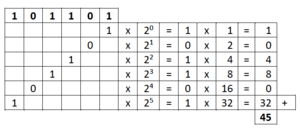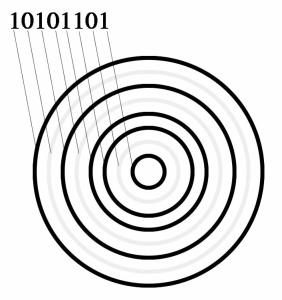From quite a young age, I am fascinated by mathematics and especially by numbers. And one of the most interesting parts of number math is that of the numeral systems.

Our common numeral system is the decimal one. Base-ten positional numeral system. Ten different digits (0,1,2,3,4,5,6,7,8,9). The position of these digits tell how much they are worth. An example.

Take the number 3028. It stands for:

 3 0 2 8 8 x 100 2 x 101 0 x 102 3 x 103 + 3 0 2 8

The decimal systems not the only system we have. Well known other systems are the Roman numeral system (ie XIV=14) but also the octal system (8 digits), the hexadecimal system (16, add A,B,C,D,E,F), sexagesimal (60) and the binary (two digit) system. The latter one forms the base of what you can see on this website.

In a binary system you only have “two fingers”. The first 16 numbers are:

0000
0001
0010
0011
0100
0101
0110
0111
1000
1001
1010
1011
1100
1101
1110
1111

It is obvious that there are all kinds of nice “rhythms” in here. The right most number alternates every number between 0 and 1, the second one from the right does the same thing but then every two numbers and so on. The system however, works the same as the decimal one. See for yourself:##### Plotting binary numbersIn the picture you see a representation of the number 10101101 (173 decimal). Every “1” is represented by a black circle. A “0” is not represented (here in very light grey, to make it clear how it works). This circle is 8 bits. That means 28 = 256 circles are possible:  00000000 until  11111111. I always want to put them in a square (if possible) so that means that here 24x24 = 16×16=256 circles are drawn. By definition every circle will be different.

You can use circles, but also squares, hexagons, triangles. Sometimes I choose to keep the form the same but then I changed the rotation binary or even the rotation and the size. Or I used protrusions or, or… There are lots of possibilities. When I think a form is interesting, I will try it out. If it looks nice, I will plot it, if not, I won’t.

##### Plotting

I use a modern plotter, the AxiDraw of the Evil Mad Scientist Laboratories. EMSL is a nice company based in California, USA. I visited it a few years ago. I use Processing to code the plots. Processing is a language, designed especially for artists so an amateur as I am, will be able to work with that too. The actual plotting is doen using the Open Source vector drawing program Inkscape. There is a nice plug-in for the AxiDraw.

##### Other plots

I sometimes experiment with other systems: random lines avoiding a circle, ternary number system plots. If you want to know something about the background, the code, anything at all, please send me an email.

Arjan van der Meij